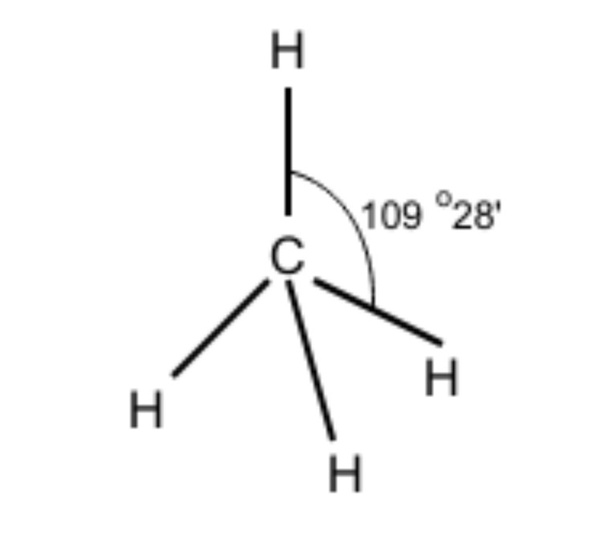Bond angle present in the methane ($CH_{ 4 }$) molecule is:A.105$^{ 0 }$B.107$^{ 0 }$C.109$^{ 0 }$D.110$^{ 0 }$Verified
147k+ views
Hint: Methane molecule has ${ sp }^{ 3 }$ hybridization. Now try to figure out the value for the standard bond angle in this hybridization.

-Let’s talk about the $CH_{ 4 }$ molecule. This is basically a combination of 1 carbon atom and 4 hydrogen atoms. However, to form this compound the central atom carbon has to complete its octet. It has 4 valence electrons and it obtains 4 more electrons from 4 hydrogen atoms. Hence, by sharing electrons between carbon and hydrogen there is a formation of a covalent bond.
-Now, we will discuss the hybridization of methane, the carbon here is ${ sp }^{ 3 }$ hybridized because one 2s orbital and three 2p orbitals in the valence shell of carbon combine to form four ${ sp }^{ 3 }$ hybrid orbitals that are of equal energy and also have equal shape. Further, four H atoms also use these four ${ sp }^{ 3 }$ hybrid orbitals of carbon to form four sigma bonds. It finally leads to the formation of the methane molecule.
-We have discussed the hybridization process. Now, determining the molecular geometry of methane should be easier for us. In methane, the four hybrid orbitals are located in such a manner so that they can decrease the force of repulsion between them. Hence, $CH_{ 4 }$ acquires a tetrahedral shape.
Hence, the ${ sp }^{ 3 }$ hybrid orbitals have a bond angle of 109$^{ 0 }$28’. We can roughly assume it to be 109$^{ 0 }$.